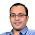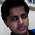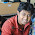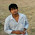### Odd Even Algorithm Puzzle

Source: http://thomer.com/riddles/

Problem:

If you have an array with random odd and even numbers, what is the most efficient algorithm you can think of to put all even numbers on one side and all odd numbers on the other side in this array? What is the complexity of your algorithm?

Update ( 21 June 2014)

Solution posted by nick, Abhishek, Khalil Sawant, Sandeep, Stainless (Ameya Ranade - CSE IITB 2009 Alumnus, Google Engineer, Facebook Engineer), Piyush Sao, Sakshi, Kaushal and Pankaj Jindal in comments! Thanks.

1.O(n) algorithm(just have to check each element once):
Start:
go from the start and check until you find odd.
Now come from the back until you find even.
Then, exchange the two number.
Go to start until start<= end

code:
a[n]//Given array
start=1;
end = n;
while(start<end)
{
if(start<end && !(a[start]%2)
start++;
if(start<end && a[end]%2)
end--;
if(start<end)
{
temp=a[start];
a[start]=a[end];
a[end]=temp;
}
}

1.Thanks Nick. Interesting solution.

2.Let's say you want to move all the odd numbers to the left side. Simply iterate with two pointers. One (p1) that points to the first even number from the left and one (p2) that iterates all the way till the end of the array. Whenever p2 encounters an odd number, it swaps with the value on p1 and proceeds (also pushing p1 one step ahead). Since each iterator can progress atmost n times and at most 1 swap occurring on each step of p2, this is an O(n) algo. Since we have to inspect every element in the array anyway, we cannot do any better.

1.Correct solution. Also, the point to appreciate is that it is O(1) space, along with O(n) time. Thanks

3.Take two indices, i and j

i goes from 0 towards end of array
j goes from n-1 towards start of array

Loop {

i skips over odd numbers, till it encounters even number
j skips over even numbers, till it encounters odd number

swap array[i] with array[j]

Repeat loop untill i crosses j
}

1.4.If you want only in terms of complexity, here is a naive O(n) algorithm: Let b be another array. First append even elements of a to b, then do one more run to append the odd elements.
You cannot do better than theta(n) because you need n operations to check whether each element is odd or even.

1.The algorithm is O(n) time. We are trying to look for O(1) space algorithm. Your solution is O(n) space.

5.An algorithm like that of partition in quicksort would do.
Initialize i to first element and j to i+1.
Maintain the invariant that all elements with index <= i have same parity and all from i to j have the other parity. Increment j to look for new elements and swap with ith element if needed (after increasing i by 1).

1.Perfect. Thanks

6.Put a pointer p1 at begin and p2 at end of array. We are going to put all odds in beginning and evens at end. if p1 has odd and p2 has even number, p1++ and p2--. if p1 has odd and p2 has odd, then p1++. if p1 has even and p2 has even, p2--. If p1 has even and p2 has odd, swap(a[p1], a[p2]) and p1++, p2--. do this till p1<p2.

1.Yep. Thanks

7.we can use partition algo

8.we can do it in O(n).
index = 0;
for(each element in array){
if(current element is even) {
swap array[index] and current element
index= index +1
}
}
after the iteration all even numbers will be in the front of array followed by all odd numbers.

1.What is the difference between current element and array[index]?

9.o(n) solution is easy. I doubt if lower complexity can be obtained, if you think of following case
1,2,3,..n-1,n;
there has to be atleast n/4 swaps.

void EvenOdd(int* A, int n)
{ //convention is to set first half to even
int left, right;
left =0 ;
right = n-1;
while(left<right)
{
while(A[left]%2==0) left++; //found a odd element on left side
while(A[right]%2==1) right--;
if(left<right) swap(A,left,right);
}
}

1.Yep. It cannot be done in less than O(n) time. We are trying to do it in O(1) space. Your solution does it in O(1) space as well :-) Thanks

10.Go through the array (say A, of size n) once to count the number of even numbers, say x.
This implies xth position now acts like a pivot.
Now, the number of odd numbers present in A to A[x-1] = number of even numbers present in A[x] to A[n]

Take two pointers, one starting from A and the other starting from A[x].
Increment 1st pointer until you find an odd number. Increment 2nd pointer until you find an even number. Then swap the values at the 2 pointers. Keep iterating on such swaps.

This is O(n) algorithm.

1.11.hash table, map evens to one index, odds to another. Runs in O(n).

12.Order(n)
--keep even numbers on left and odd ones on right
Algorithm:
even_pointer=1
odd_pointer=n
main_loop:
If even, increment the even_pointer by 1
If odd:
odd_loop:
Read the element at the odd_pointer.
if odd: reduce the odd_pointer. continue

1.13.O(n), where n is the size of the array.

Without loss of generality, lets assume we want to keep the odd numbers on the left hand side and the even numbers on the right hand side of the array.

Let i=0 and j=n-1 be two variables. (assuming 0-based indexing)
Start from the left and increment i till you reach an array index such that array[i] is even, then start from the right and decrement the variable j till you reach an array index such that array[j] is odd. Then swap the elements array[i] and array[j], increment i and decrement j. Do this until i becomes j.

1.14.without loss of generality, lets assume a is odd, Keep this as a block of size 1. Start going through the a[i] 's for i = 1, 2, 3, ..., n-1
1. If a[i] is odd, stick it at the end of block (This increases block size by 1).
2. If even, swap leftmost (0 th) element of odd block with a[i] (This does nt change block size).

So it is O(n) algorithm.